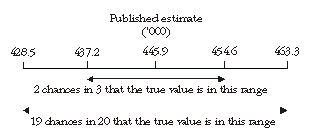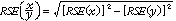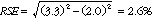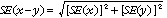6265.0 - Underemployed Workers, Australia, Sep 2010
ARCHIVED ISSUE Released at 11:30 AM (CANBERRA TIME) 08/03/2011
Page tools:Print All

TECHNICAL NOTE DATA QUALITY

INTRODUCTION

1 Estimates in this publication are based on information obtained from occupants of a sample of dwellings, and are subject to sampling variability. That is, they may differ from those estimates that would have been produced if all dwellings had been included in the survey. One measure of the likely difference is given by the standard error (SE), which indicates the extent to which an estimate might have varied by chance because only a sample of dwellings was included. There are about two chances in three (67%) that a sample estimate will differ by less than one SE from the number that would have been obtained if all dwellings had been included, and about 19 chances in 20 (95%) that the difference will be less than two SEs. Another measure of the likely difference is the relative standard error (RSE), which is obtained by expressing the SE as a percentage of the estimate.

2 Due to space limitations, it is impractical to print the SE of each estimate in the publication. Instead, a table of SEs is provided to determine the SE for an estimate from the size of that estimate ( see table T1). The SE table is derived from a mathematical model, referred to as the 'SE model', which is created using data from a number of past Labour Force Surveys. It should be noted that the SE model only gives an approximate value for the SE for any particular estimate, since there is some minor variation between SEs for different estimates of the same size.

CALCULATION OF STANDARD ERROR

3 An example of the calculation and the use of SEs in relation to estimates of persons is as follows. Table 4 shows the estimated number of female underemployed part-time workers was 445,900. Since this estimate is between 300,000 and 500,000, table T1 shows that the SE for Australia will lie between 7,300 and 9,200 and can be approximated by interpolation using the following general formula:4 Therefore, there are about two chances in three that the value that would have been produced if all dwellings had been included in the survey will fall within the range 437,200 to 454,600 and about 19 chances in 20 that the value will fall within the range 428,500 to 463,300. This example is illustrated in the following diagram.5 In general, the size of the SE increases as the size of the estimate increases. Conversely, the RSE decreases as the size of the estimate increases. Very small estimates are thus subject to such high RSEs that their value for most practical purposes is unreliable. In the tables in this publication, only estimates with RSEs of 25% or less are considered reliable for most purposes. Estimates with RSEs greater than 25% but less than or equal to 50% are preceded by an asterisk (e.g.*3.2) to indicate they are subject to high SEs and should be used with caution. Estimates with RSEs of greater than 50%, preceded by a double asterisk (e.g.**0.3), are considered too unreliable for general use and should only be used to aggregate with other estimates to provide derived estimates with RSEs of less than 25%.

MEANS AND MEDIANS

6 The RSEs of estimates of mean duration of insufficient work, median duration of insufficient work and mean preferred number of extra hours are obtained by first finding the RSE of the estimate of the total number of persons contributing to the mean or median ( see table T1) and then multiplying the resulting number by the following factors:
• mean duration of insufficient work: 1.6
• median duration of insufficient work: 2.5
• mean preferred number of extra hours: 0.7

7 The following is an example of the calculation of SEs where the use of a factor is required. Table 4 shows that the estimated number of male underemployed part-time workers was 288,000 with a median duration of insufficient work of 26 weeks. The SE of 288,000 can be calculated from table T1 (by interpolation) as 7,200. To convert this to an RSE we express the SE as a percentage of the estimate or 7,200/288,800 = 2.5%.

8 The RSE of the estimate of median duration of insufficient work is calculated by multiplying this number (2.5%) by the appropriate factor shown in paragraph 7 (in this case 2.5): 2.5 x 2.5 = 6.3%. The SE of this estimate of median duration of insufficient work is therefore 6.3% of 26, i.e. about 2 (rounded to the nearest whole week). Therefore, there are two chances in three that the median duration of insufficient work for males that would have been obtained if all dwellings had been included in the survey would have been within the range 24-28 weeks, and about 19 chances in 20 that it would have been within the range 22-30 weeks.

PROPORTIONS AND PERCENTAGES

9 Proportions and percentages formed from the ratio of two estimates are also subject to sampling errors. The size of the error depends on the accuracy of both the numerator and the denominator. A formula to approximate the RSE of a proportion is given below. This formula is only valid when x is a subset of y.10 Considering the example from paragraph 3, of the 445,900 female underemployed part-time workers, 179,300 or 40.0% had insufficient work for 52 weeks and over. The SE of 179,300 may be calculated by interpolation as 5,900. To convert this to an RSE we express the SE as a percentage of the estimate, or 5,900/179,300 = 3.3%. The SE for 445,900 was calculated previously as 8,700, which converted to an RSE is 8,700/445,900 = 2.0%. Applying the above formula, the RSE of the proportion is:11 Therefore, the SE for the proportion of females who have a current period of insufficient work of 52 weeks or more is 1.0 percentage points (=(40.0/100)x2.6). Therefore, there are about two chances in three that the proportion of females who have a current period of insufficient work of 52 weeks or more was between 39.0% and 41.0% and 19 chances in 20 that the proportion is within the range 38.0% to 42.0%.

DIFFERENCES

12 Published estimates may also be used to calculate the difference between two survey estimates (of numbers or percentages). Such an estimate is subject to sampling error. The sampling error of the difference between two estimates depends on their SEs and the relationship (correlation) between them. An approximate SE of the difference between two estimates (x-y) may be calculated by the following formula:13 While this formula will only be exact for differences between separate and uncorrelated characteristics or subpopulations, it is expected to provide a good approximation for all differences likely to be of interest in this publication.

STANDARD ERRORS

 STANDARD ERRORS OF ESTIMATESNSW Vic. Qld SA WA Tas. NT ACT SE RSE Size of Estimate (persons) no. no. no. no. no. no. no. no. no. % 100 320 310 240 190 240 120 90 110 120 120.0 200 440 420 350 260 320 170 130 180 210 105.0 300 520 490 430 310 380 210 160 230 290 96.7 500 640 600 550 380 460 260 210 300 420 84.0 700 730 680 640 430 530 300 250 330 530 75.7 1,000 840 780 750 500 600 340 290 370 670 67.0 1,500 990 910 890 580 710 400 340 400 860 57.3 2,000 1 110 1 020 1 000 650 790 440 380 430 1 010 50.5 2,500 1 200 1 100 1 100 700 850 450 400 450 1 150 46.0 3,000 1 300 1 200 1 150 750 900 500 450 500 1 250 41.7 3,500 1 400 1 250 1 250 800 950 500 450 500 1 350 38.6 4,000 1 450 1 350 1 300 850 1 000 550 450 500 1 450 36.3 5,000 1 600 1 450 1 400 900 1 100 600 500 550 1 650 33.0 7,000 1 800 1 650 1 600 1 000 1 250 650 600 650 1 900 27.1 10,000 2 050 1 850 1 750 1 150 1 400 750 800 750 2 200 22.0 15,000 2 400 2 150 2 000 1 300 1 650 950 1 100 950 2 550 17.0 20,000 2 650 2 400 2 150 1 450 1 800 1 100 1 400 1 100 2 850 14.3 30,000 3 100 2 800 2 500 1 750 2 100 1 350 1 950 1 350 3 200 10.7 40,000 3 400 3 100 2 750 2 000 2 300 1 650 2 450 1 650 3 500 8.8 50,000 3 700 3 350 3 050 2 200 2 550 1 850 2 950 1 850 3 750 7.5 100,000 4 700 4 400 4 150 3 300 3 750 2 650 5 100 2 450 4 750 4.8 150,000 5 550 5 350 5 100 4 250 4 950 3 150 7 000 2 750 5 500 3.7 200,000 6 350 6 250 5 950 5 000 5 950 3 550 8 750 2 950 6 150 3.1 300,000 8 000 7 950 7 550 6 150 7 450 4 050 11 950 3 100 7 300 2.4 500,000 11 200 11 050 10 200 7 700 9 500 4 700 . . 3 100 9 200 1.8 1,000,000 16 650 16 850 14 550 9 800 12 100 5 350 . . . . 12 950 1.3 2,000,000 22 450 24 900 19 600 11 650 14 000 . . . . . . 18 800 0.9 5,000,000 28 600 39 800 26 400 13 150 14 600 . . . . . . 31 400 0.6 10,000,000 30 650 54 900 30 900 . . . . . . . . . . 41 900 0.4 15,000,000 . . . . . . . . . . . . . . . . 47 250 0.3 . . not applicable